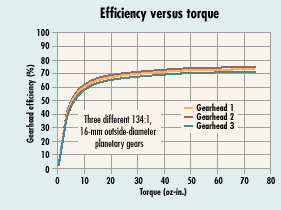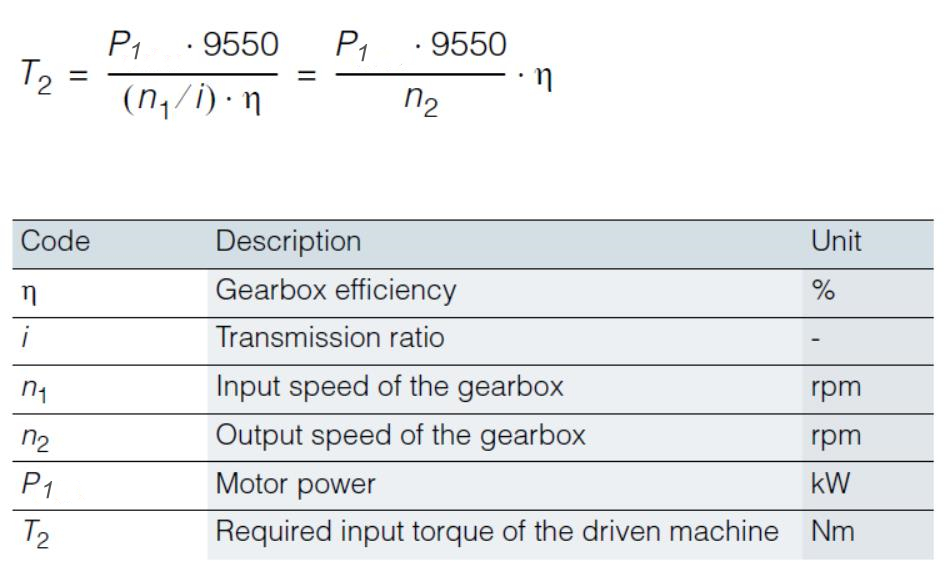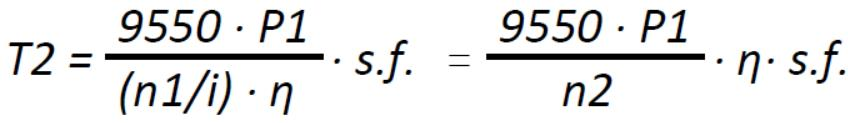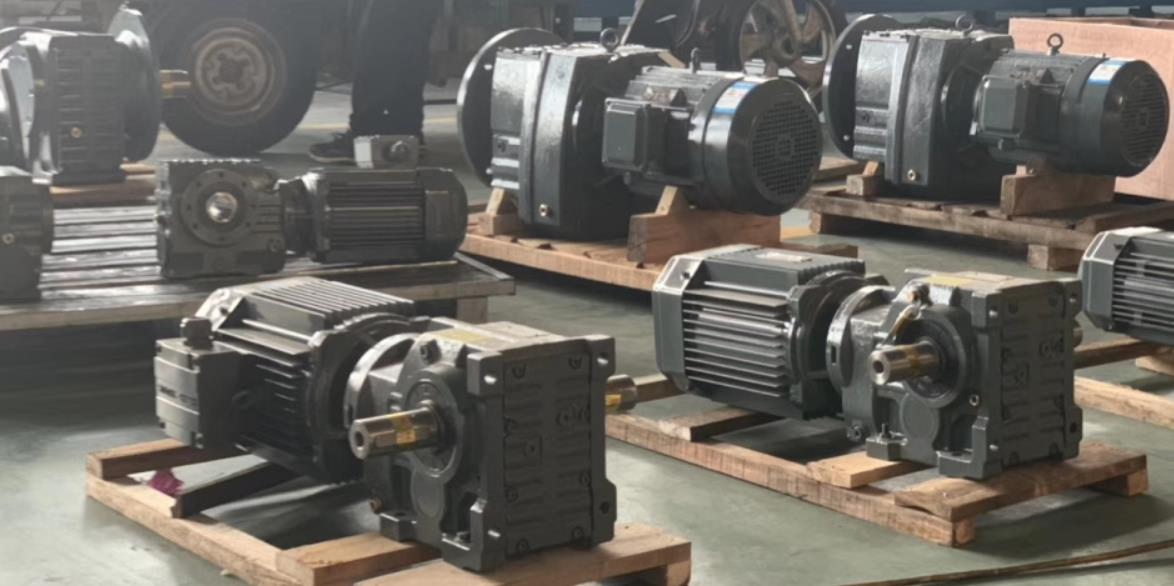SDT Transmission customers usually want to know how to calculate gearbox torque when they select industrial gear reducer models by equipment actual working condition, they always have the following problems: How to calculate industrial gearbox torque? how to match industrial speed reducer with motor power? especially for worm drive gearbox, helical gear motor and cycloidal gear reducer, the output torque can be transmitted continuously through the output shaft of the gearbox, with the gear unit operated under a service factor (f.s.) = 1.0. the output torque is one of the most important technical parameters to motor and gearbox calculations, please contact us if you need gearbox torque calculation formula pdf.  Here introduce gearbox torque calculation and guidance to motor power:How to calculate gearbox output torque? ( gearbox output torque calculation formula)

Ratio = number of revolutions of the motor output speed/gearbox output revolutions (“Ratio” also known as ” transmission ration”)

Gearbox Output Torque Calculation Formula• You can get the gearbox output torque if you know motor power(kW or HP, for short), ratio(i), and service factor(f.s.), the gearbox torque formula is as follows:

Gearbox torque calculation formula:

Gearbox Output Torque=9550×Motor Power<Kw>/(Input Speed of rated power’s motor<R/min or RPM> / Total ratio)×Service factor x Gearbox transmission efficiencyT2 = 9550 x P1/(n1 / i) x f.s. x η = 9550 x P1 / n2 x f.s. x η

 Code Description Unit T2 Gearbox output torque N.m (Kgs.m) P1 Input Power Kw n1 Input speed r/min (RPM) i Total ratio – n2 Output speed r/min (RPM) f.s. Service factor – η Gearbox transmission efficiency %

• Know Torque on gearbox output shaft/bore, the output speed of gearbox and service factor, how to calculate motor power?

Motor Power(HP or Kw)=Torque (N.m) /9550×Input Speed of motor Power(Kw) / Ratio / Service factor

The industrial gearbox is a relatively precise machine, its purpose is to decrease motor speed, increase output torque.

The following situation is an example of the gearbox torque calculation, Customer can refer to the below information:The gear reducer output shaft is one position of output torque performance on the Gearmotor.

Question:
Gear speed reducer with 0.75Kw input power, transmission ratio 1:30, default service factor(f.s.) is 1.0, and gearbox transmission efficiency(η):100%, 4 pole AC induction motor with 1440 rpm, How to calculate the gearbox output torque(T2)? What are the units of output torque?  How heavy goods can be lifted by this industrial Gearmotor?

0.75Kw( geared motor power) x9550x gearbox transmission efficiency  x30 / input speed x service factor = gearbox output torque.
If the AC induction motor is 4 Pole AC asynchronous motor: 1380 rev/min. (also short for : r/min; RPM):
Gearbox output torque= 0.75x9550x100% x30 / 1380 x1.0 = 155.7 (N.M), it means this industrial gear motor can lift 155.7 N.m (15.877 kgs.m) goods.

If you need to know the output torque of the gear reducer, only substitute it into the rotation efficiency, parameter values of motor input speed. calculate the above gearbox output torque(T2), then you can refer to SDT Transmission gear motor select model table, get the suitable gearbox model.

The torque unit is N.m (Newton · meters) or kilogram. meters (Kg · m).
Gear Reducer Torque = 9550 * Motor power / Rated Power motor’s input revolution*Ratio* gear reducer efficiency.
The above calculation is the torque equation.
Motor power = torque ÷ 9550 × Rated power‘s motor input speed /Ratio /service factor.

Here useful converted relationship to Output torque’s unit  :

 100 N.m = 10.1972 Kg.m 100 N.m = 885.07457 9349 Ibs in 100N.m = 885.07458 ibf.in 100 N/m = 0.5710147 ib/in

 Service factor(f.s.):
The service factor is also called the using factor or safety factor; especially for users who select the gearbox, the gearbox’s use factor should be determined in combination with other factors like working conditions and driven machinery. (generally> 1.0, it means gear reducer capacity> the motor capacity). Ensure that it has a margin to the gearbox rated mechanical energy and thermal energy.

 Gearbox transmission efficiency(η):
The definition of gearbox transmission efficiency: refers to the ratio of the output power (or output torque) to the rated input power (or input torque multiplied by the transmission ratio) when the rated input speed reaches the rated input power (or input torque). The efficiency of the gearbox is determined in part by the gear teeth, the rolling-contact bearing friction, and the shaft seal friction.It can be measured on a dedicated test bench, and the methods include the loss power method and the direct power measurement method. Different gear arrangement gearbox with vary transmission efficiency:
Single-stage planetary gear:97% , two-stage planetary gear:94%, three-stage planetary gear: 91%.
1 stage Archimedes worm gearbox: 85%, 2 stages Archimedes worm gearbox:72%.
One stage helical gear unit: 95%, two-stage helical gear unit:90%.

 Gearbox output torque(T2):
Gearbox output torque refers to the actual working output torque. after the user calculates gearbox output torque, according to the SDT Transmission catalogue, not only the gearbox rated output torque exceed than actual working output torque, but also consider the overload capacity of the drive motor and the required maximum operating torque in practice.
Theoretically, the required maximum working torque must be less than the gearbox rated output torque. in particular, some tough working conditions must strictly abide by this criterion.

Do you have more queries if considering service factor, gearbox transmission efficiency, etc? chat to SDT Transmission online, in order to get a quick reply, you also can fill in the table.